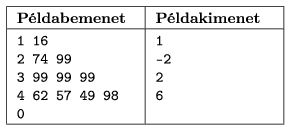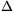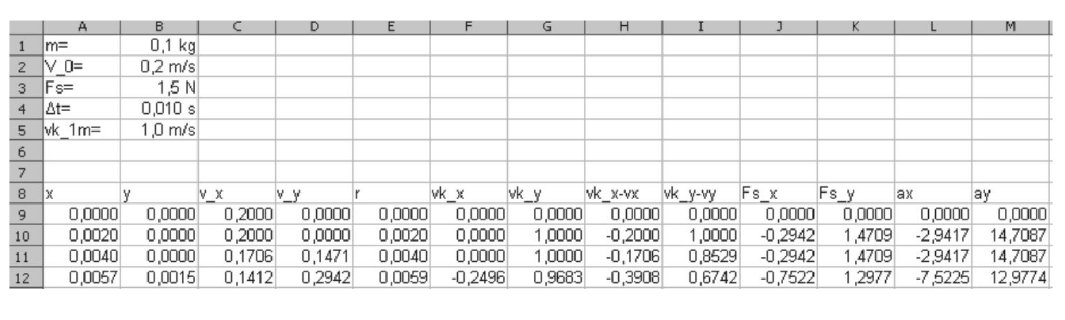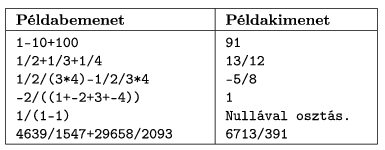Mathematical and Physical Journal
for High Schools
Issued by the MATFUND Foundation
 Already signed up? New to KöMaL?

# KöMaL Problems in Informatics, November 2008

Show/hide problems of signs:## Problems with sign 'I'

Deadline expired on December 15, 2008.

I. 196. Using at least 12 digits of precision, find the real solution of equation sin x+cos x+tan x+cot x=x which has the smallest absolute value.

The root should be submitted together with a short documentation (i196.txt, i196.pdf, ...) and any other auxiliary materials (such as figures, source codes or tables) created by the Contestant.

(10 pont)

statistics

I. 197. We are buying N different items in a shop, not necessarily at once, but during several purchases. From March 1, 2008 in Hungary, the final Forint (HUF) sum of a purchase has to be rounded off to the nearest integer multiple of 5 HUF. Knowing the prices of items, write a program to decide which items should be bought during which purchase such that our loss due to these rounding-offs is minimized.

The number of products and their prices are read from the standard input. Each line of the input contains a list of items to be purchased: the number N of items, then their prices a1,...,aN (positive integers, separated by a space). The total sum in each line is at most. The end of input is denoted by a line containing a single 0''.

The output is written to the standard output. Each line of the output corresponds to a line in the input, and contains a single integer: our gain or loss in HUF due to the rounding-offs if optimal purchases are made.

In the table, Példabemenet'' is the input and Példakimenet'' is the output.The source code of your program (i197.pas, i197.cpp, ...) together with a short documentation (i197.txt, i197.pdf, ...) should be submitted, also containing a brief description of your solution and the name of the developer environment to use for compiling the source code.

(10 pont)

solution (in Hungarian), statistics

I. 198. Using a spreadsheet application, simulate the motion of a point mass lying on a rotating disc.

The disc is horizontal, the rotation is uniform and anticlockwise. The axis of rotation is vertical and it coincides with the axis of symmetry of the disc. The peripheral velocity vk of any point of the disc is perpendicular to the radius, and its magnitude is directly proportional to the distance from the center.

The object having mass m is initially located at the center of the disc and it has initial velocity V0. The object then moves horizontally on the disc only under the influence of the constant slip friction force Fs. The direction of this force is opposite to the velocity of the object relative to the disc.

The simulation is performed by choosing a suitable time-stept, in which all physical quantities can be considered as constants. The state of the system at any time instant determines the state of the system after timet according to the rules below:the position coordinates of the object x and y should be increased byt multiplied by the previous velocity coordinates vx and vy;the distance r of the object from the center is computed together with the vkx and vky components of the peripheral velocity of the given point of the disc;the difference of the velocity vector of the object and that of the disc is computed, since this gives the direction of the friction force;components of the friction force are computed, from which components of the acceleration vector are obtained;components of the acceleration multiplied byt give the change of velocity: velocity in the next simulation step should be increased by these values.

The mass of the object, its initial velocity, the magnitude of the friction force, the time-step and the peripheral velocity of a point of the disc (located by 1 m from the center) are given in the first 5 lines of the sheet, according to the example. The following lines of the sheet should contain the quantities describing the motion of the object in at least 300 simulation steps. The motion of the object should be depicted on an appropriate diagram.Your worksheet (i198.xls, i198.ods, ...) together with a short documentation (i198.txt, i198.pdf, ...) should be submitted, also containing a brief description of your solution and the name and version number of the spreadsheet application used.

(10 pont)

solution (in Hungarian), statistics## Problems with sign 'S'

Deadline expired on December 15, 2008.

S. 39. Write a program that implements a calculator. The calculator should be able to evaluate expressions containing the four basic arithmetic operations (+,-,*,/), integers and parentheses. Operations should have the usual precedence: multiplication and division have the same priority, further, addition and subtraction have the same priority, but multiplication and division are evaluated first. If there are no parentheses, and several operations of the same precedence are present, evaluation is performed from left to right, for example e-f-a/b/c*d=(e-f)-(((a/b)/c)*d).

The expressions to be evaluated are found in the input file, whose name is the first command line argument of your program, while results are written into the output file, whose name is the second command line argument. Each line of the input file contains an expression. The corresponding single line in the output file should contain the evaluation of this expression: the exact result should be given as a fraction or integer in its simplest form. Issue a warning of Division by 0'' when necessary.

The length of each input line is at most 250 characters and each expression is syntactically correct. You can further assume that during the computations each intermediate result (in its simplest form) can be represented as a ratio of 16-bit integers. (However, temporarily it may be necessary to use 32-bit integers to avoid overflow.)

In the example, Példabemenet'' is the input, Példakimenet'' is the corresponding output, while Nullával osztás'' is division by 0.The source code of your program (s39.pas, s39.cpp, ...) together with a short documentation (s39.txt, s39.pdf, ...) should be submitted, also containing a brief description of your solution and the name of the developer environment to use for compiling.

(10 pont)

statistics

\$Var(Body)